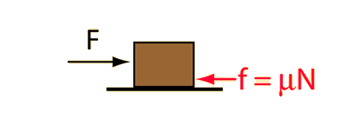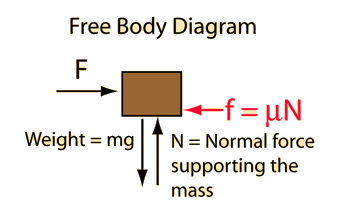# Constant Acceleration MotionIn the standard model of friction, the frictional resistance is given by the coefficient of friction times the normal force.For this case the normal force is just the weight of the object.
Application of Newton's second law to a single mass.
If an applied force F=N is opposed by friction with friction coefficient mu = acting on mass m=kg then the frictional resistance force is N and the net force is = N. Newton's second law becomes:

### Net force = mass x acceleration

Net force = N = kg x m/s²

You may edit any of the data. When finished with data entry, click on the quantity you desire to calculate in the formula above. Quantities will not be forced to be consistent until you click on the desired quantity.

In U.S. common units this becomes:
Net force = lb = slug x ft/s²

In this system the weight on the earth's surface islbs.

### Remove friction

Index

Newton's laws

Standard mechanics problems

 HyperPhysics***** Mechanics R Nave
Go Back